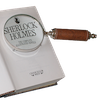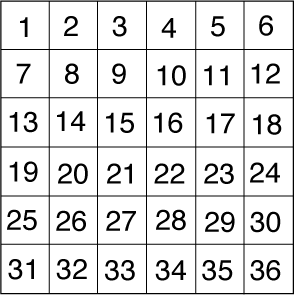#### You may also like### Number Round Up

Arrange the numbers 1 to 6 in each set of circles below. The sum of each side of the triangle should equal the number in its centre.### Number Detective

Follow the clues to find the mystery number.### Grouping Goodies

Pat counts her sweets in different groups and both times she has some left over. How many sweets could she have had?

# One of Thirty-six

## One of Thirty-six

Can you find the chosen number from this square using the clues below?1. The number is odd.
2. It is a multiple of three.
3. It is smaller than $7\times 4$.
4. Its tens digit is even.
5. It is the greater of the two possibilities.

You might like to print off this sheet of the problem.

### Why do this problem?

One of Thirty Six encourages pupils to apply their knowledge of number properties in a logical way. The challenge in this problem is to decide in which order the information in the clues is useful.

### Possible approach

A nice way to start a lesson which focused on this problem would be to play a version of the "What's my rule?" game. Decide on a number property, for example odd numbers, and draw a large circle on the board. Explain to the children that they have to work out your rule by suggesting just, for example, ten numbers. If the number they suggest fits your rule, you write it in the circle. If not, write it outside the circle. After ten suggestions, can they work out the rule? (You might have to alter the number of suggestions they're allowed!) Play this game a few times, perhaps asking individuals to come to the board and be the one to choose the rule.

You could then look at this problem all together and ask pairs of children to try and solve it. Emphasise that after a specified length of time you will be asking them HOW they went about solving it rather than just wanting to know the answer. It would be useful for each pair to have a copy of the problem - you could print off this sheet. It might be worth stopping them after just a few minutes to share good ways of keeping track of what they're doing. Some children might suggest crossing out or circling numbers on the grid, for example.

Once the children have worked on this problem (and possibly the extension too), the key point to bring out in a whole class discussion is the idea that the first clue was not necessarily the most useful to start with. Invite pairs to describe how they found the solution, emphasising where choices were made as to which clue they used next.

### Key questions

How did you go about finding the solution?